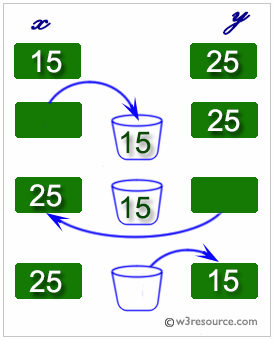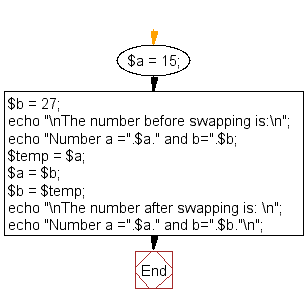﻿ PHP Exercise: Swap two variables - w3resource# PHP Exercises : Swap two variables

## PHP : Exercise-31 with Solution

Write a PHP program to swap two variables.

PHP: Swapping two variables

Swapping two variables refers to mutually exchanging the values of the variables. Generally, this is done with the data in memory.

The simplest method to swap two variables is to use a third temporary variable :

```define swap(a, b)
temp := a
a := b
b := temp
```Sample Solution: -

Code : Swap two numbers using a third variable

PHP Code:

``````<?php
\$a = 15;
\$b = 27;

// Swap Logic

echo "\nThe number before swapping is:\n";
echo "Number a =".\$a." and b=".\$b;

\$temp = \$a;
\$a = \$b;
\$b = \$temp;

echo "\nThe number after swapping is: \n";
echo "Number a =".\$a." and b=".\$b."\n";
?>
```
```

Code: Swap two variables value without using third variable

``````<?php
\$a = 15;
\$b = 276;
echo "\nBefore swapping:  ". \$a . ',' . \$b;
list(\$a, \$b) = array(\$b, \$a);
echo "\nAfter swapping:  ". \$a . ',' . \$b."\n";
?>
```
```

Sample Output:

```The number before swapping is:
Number a =15 and b=27
The number after swapping is:
Number a =27 and b=15
```

Flowchart:PHP Code Editor:

Have another way to solve this solution? Contribute your code (and comments) through Disqus.

What is the difficulty level of this exercise?

Test your Programming skills with w3resource's quiz.

﻿

## PHP: Tips of the Day

Why shouldn't I use mysql_* functions in PHP?

The MySQL extension:

• Is not under active development
• Is officially deprecated as of PHP 5.5 (released June 2013).
• Has been removed entirely as of PHP 7.0 (released December 2015)
• This means that as of 31 Dec 2018 it does not exist in any supported version of PHP. If you are using a version of PHP which supports it, you are using a version which doesn't get security problems fixed.
• Lacks an OO interface
• Doesn't support:
• Non-blocking, asynchronous queries
• Prepared statements or parameterized queries
• Stored procedures
• Multiple Statements
• Transactions
• The "new" password authentication method (on by default in MySQL 5.6; required in 5.7)
• Any of the new functionality in MySQL 5.1 or later

Since it is deprecated, using it makes your code less future proof.

Lack of support for prepared statements is particularly important as they provide a clearer, less error-prone method of escaping and quoting external data than manually escaping it with a separate function call.

Ref : https://bit.ly/2BIIsF0